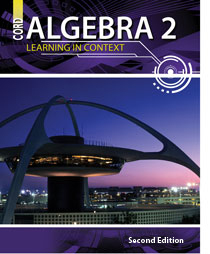# Algebra 2 - Second Edition

## Chapter 7: Rational Functions

Some links are repeated for use with more than one lesson.

### These links are for use with the entire chapter.

http://www.regentsprep.org/Regents/math/algtrig/ATO2/indexATO2.htm

http://www.xpmath.com/careers/topicsresult.php?subjectID=2&topicID=6

### 7.1 Multiplying and Dividing Rational Expressions

http://www.algebralab.com/lessons/lesson.aspx?file=Algebra_rational_operations.xml

http://www.regentsprep.org/Regents/math/algtrig/ATO2/indexATO2.htm
This series of pages contains lessons on multiplying and dividing rational expressions.

### 7.2 Adding and Subtracting Rational Expressions

This series of pages uses several examples to demonstrate adding and subtracting rational expressions.

### 7.3 Complex Fractions

http://www.regentsprep.org/regents/math/algtrig/ATV2/indexATV2.htm

http://www.purplemath.com/modules/compfrac.htm
This series of pages uses several examples to demonstrate handling complex fractions.

### 7.4 Graphing Rational Functions

http://www.purplemath.com/modules/grphrtnl.htm
This series of pages uses several examples to demonstrate how to graph rational functions.

http://www.prenhall.com/divisions/esm/app/graphing/ti83/Graphing/
setting_windows/Examples/rational/rational_example.html

This page contains an explanation of graphing rational functions on a TI-83.

http://hotmath.com/help/gt/genericalg2/section_7_1.html

### 7.5 Solving Rational Equations

http://www.purplemath.com/modules/solvrtnl.htm
This series of pages uses several examples to demonstrate solving of equations with variables in the denominator.

http://www.regentsprep.org/Regents/math/algtrig/ATE11/RationalEquationsLes.htm
This page presents strategies for solving rational equations, stressing the importance of checking the results.

### 7.6 Direct, Inverse, and Joint Variation

http://hotmath.com/help/gt/genericalg2/section_7_2.html